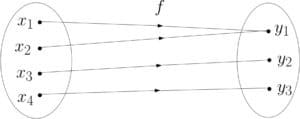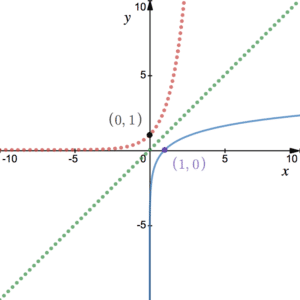# Inverse Functions and Composite Functions

Before we take a look at inverse functions and composite functions, we must know the difference between a mapping and a function and how to define the domain and range of a function.A mapping is any algebraic equation or graph that transforms one set of numbers to another. The diagram shows an example of a many-to-one mapping. In a similar way, we also get one-to-many and one-to-one mappings. For a mapping to be a function, it must be either one-to-one or many-to-one. For the function to be invertible it must be one-to-one only. See more on Inverse Functions below.

### Domain & Range

We consider the DOMAIN of a function to be the full set of values that we can successfully enter into the function. Some functions to be aware of:

• SQUARE ROOTING – we can only square root non-negative numbers (this includes 0)
• LOGARITHMS – we can only log positive numbers (this doesn’t include 0) – see more on logs
• DIVISION BY 0 – we can only divide by numbers that are not zero.

The RANGE of a function is the full set of values that we can get out of the function. It follows that we determine the range from the domain. Note that we may restrict the domain, i.e. we may exclude some values from the domain that are otherwise fine to have. This will then have an effect on the range which will also be restricted. See Example 1 for more on domain, range and restrictions.

## Inverse Functions

As above, if a function is one-to-one, then it is invertible. We can think of the inverse function as the function that performs the reverse operations. For a one-to-one function $f(x)$, the inverse function is $f^{-1}(x)$. For example, if $f(x)=2x+5$ then $f^{-1}(x)=\frac{x-5}{2}$. Note that we perform the reverse operations in the reverse order.

The inverse function for more complicated functions can be found as follows:

1. Write the function in explicit form, i.e. $y$ equals expression in terms of $x$.
2. Swap the $x$s for $y$s and the $y$s for $x$s.
3. Make $y$ the subject of this new expression.
4. Label it as $f^{-1}(x)$. See Example 2.

Note that if we get the same function back when we find its inverse, the function is known as a self-inverse function. The reciprocal function (1/x) is an example of a self-inverse function.The graphs of $f(x)$ and $f^{-1}(x)$ are reflections of one another across the line $y=x$. It follows that the domain of the inverse function is the range of the original function. Similarly, the domain of the original is the range of the inverse function. The diagram shows the graphs of $y=\ln(x)$ (blue solid),  $y=e^x$ (red dotted) and $y=x$ (green dotted). Since $\ln(x)$ snd $e^x$ are inverses of one another, their graphs are reflections of one another in the line $y=x$. See Example 3 for another example.

Note that the graph of a self-inverse function will be the same when reflected in the line $y=x$. For example, the graph of $y=\frac{1}{x}$ has reflectional symmetry in the line $y=x$.

## Composite Functions

Composite functions are where we apply a function on top of another function. We often call these chains. For example, $y=\sin(x+5)$ is a composite function since we can identify two separate functions: the function where we add 5, then the function where we sine the result.

Given two functions $f(x)$ and $g(x)$. We can define the composite functions $fg(x)$ and $gf(x)$. These are sometimes denoted $f\circ g(x)$ and $g\circ f(x)$ respectively. Note that we can think of $fg(x)$ as $f(g(x))$, that is, we apply $f$ after we apply $g$ to $x$. Hence, we find the function of $fg(x)$ by applying $g$ to $x$ and applying $f$ afterwards. Usually the function $gf(x)$, which we find by applying $f$ first then $g$, is quite different from the function $fg(x)$. This is because $f(x)$ and $g(x)$ are generally different functions. See Example 3.

Note that $ff^{-1}(x)=f^{-1}f(x)=x$. We can see that if we apply $f$ to $x$ then apply the inverse of $f$, or vice versa, we will get the original $x$ value back.

## Example of Inverse Functions and Composite Functions

1. What is the domain and range for the function $f:x\mapsto\sqrt{7-3x}$?
2. Find the domain and range for the function $g(x)=2\ln(5x+2)+4$.
3. What is the range of the function $h(x)=6-\frac{8}{4-x}$ with restricted domain $\left\lbrace x\in{\mathbb R}:x\geq 5\right\rbrace$?

Find the inverse functions for $f(x)=\frac{2x}{x-3}$, $x\ne 3$.

We define the functions $f$ and $g$ as follows:

$f:x\rightarrow 2^{-x}, \hspace{10pt}x\in{\mathbb R}$

$g:x\rightarrow 5(x-3), \hspace{10pt}x\in{\mathbb R}$.

1. State the range of $f(x)$. State the domain of $f^{-1}(x)$.
2. Sketch $g(x)$ and $g^{-1}(x)$ on the same graph.
3. Find the function $gf(x)$.
4. Solve the equation $fg(x)=16$.